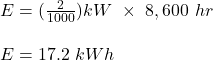## A clock draws 2.0 watts of power to operate and is in operation 8,600 hours per year. The energy usage of the clock, in kWh per year, is clo

Question

A clock draws 2.0 watts of power to operate and is in operation 8,600 hours per year. The energy usage of the clock, in kWh per year, is closest to

in progress 0
2 months 2021-07-30T22:53:53+00:00 1 Answers 2 views 0

the energy usage of the clock per year is 17.2 kWh

Explanation:

Given;

power drawn by the clock, P = 2 W

duration of the clock’s operation, t = 8,600 hours per year

The energy usage of the clock per year is calculated as;

E = PtTherefore, the energy usage of the clock per year is 17.2 kWh